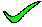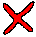You would rewrite W with a better key (c,b), since c->a means that c is a better key than a. After that the normalisation is straightforward, and the result is that shown here.

closeThis set of relations solves nothing, and does not change the fact that W is still not in BCNF.

closeThis set of relations are actually valid when considered seperately, but the resulting query is suboptimal and could result in spurious values (if 'a' contained duplicate values).

closeYou cannot delete a directly from the composite key of W, as this would be an illegal operation. You must have 'a' in the key, either directly or indirectly. Note that as c gives a, you could substitute the attribute c every time you use a in a key.

closeYou would rewrite W with a better key (c,b), since c->a means that c is a better key than a. After that the normalisation is straightforward.

close

 A B C D E TELL ME NEXT INDEX

If the relation W was normalised to BCNF, select the best resulting relationsof that process.

``` W(a,b,c,d,e)
c -> a
```
1. ``` W(c,b,d,e)
W1(c,a)
```
2. ``` W(a,b,c,d,e)
W1(c,a)
```
3. ``` W(a,b,d,e)
W1(c,a)
```
4. ``` W(b,c,d,e)
W1(c,a)
```
5. None of the above